# Difference between revisions of "Whitney mapping"

Letbe a compact Hausdorff space. The hyperspace ofis denoted by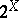; the subspace of, consisting of all sub-continua ofis denoted by. A Whitney mapping ofis a real-valued continuous function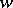on(or on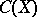) with the following properties:

1)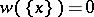for each;

2) ifandare in the domain ofand ifis a proper subset of, then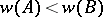.

A set of type, for, is called a Whitney level.

The existence of a Whitney function onimplies thatis a-subset of its hyperspace, which in turn implies thatis metrizable (cf. also Metrizable space). One of the simplest constructions of a Whitney mapping for a compact metrizable spaceis as follows. Letbe a countable open base of, and, for each pair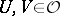such that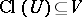, fix a mapping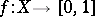which equalsonandoutside. Enumerate these functions as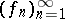and let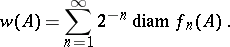Below it is assumed that all spaces are metric continua. Whitney functions have been developed as a fundamental tool in continua theory. Their first use in this context was involved with order arcs in, and led to a proof thatis acyclic and that bothandare even contractible ifis Peanian (1942). Later on they became a subject of study in their own right.

A Whitney property is a topological property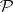such that if a metric continuumhas, then so does each Whitney level of it in. Examples are: being a (locally connected) continuum; being a hereditarily indecomposable continuum; being a (pseudo-) arc or a circle; etc. Counterexamples are: being contractible; being acyclic in Alexander–Čech cohomology; being homogeneous; being a Hilbert cube.

Whitney functions were introduced by H. Whitney [a4] in 1933 in a different context. They were first used by J.L. Kelley [a2] to study hyperspaces. Every metric continuum can occur as a Whitney level [a1]. For an account of continua theory see [a3].